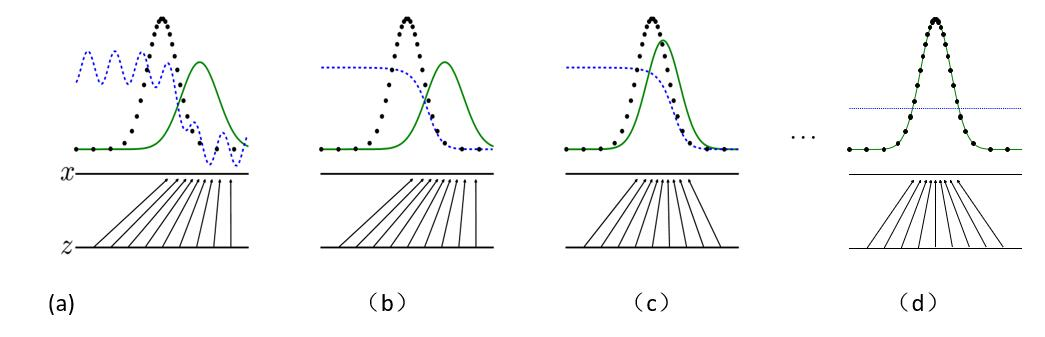# [翻译]生成对抗网络

(在苹果系统下，如果文章中的图片不能正常显示，请升级Safari浏览器到最新版本，或者使用Chrome、Firefox浏览器打开。)

Ian J. Goodfellow, Jean Pouget-Abadie, Mehdi Mirza, Bing Xu, David Warde-Farley,

Sherjil Ozair, Aaron Courville, Yoshua Bengio§

Departement d’informatique et de recherche op´ erationnelle´

Universite de Montr´ eal´

Montreal, QC H3C 3J7´

## 2 相关工作

$$\mathop{lim}\limits_{\sigma \rightarrow 0}{ \bigtriangledown _x \mathbb{E}_{ \epsilon \sim N(0,\sigma^2 I)}f(x+\epsilon) }=\bigtriangledown_x f(x).$$

## 3 对抗网络

–$$\min\limits_{G} \max\limits_{D} V(D,G)=\mathbb{E}_{x \sim p_{data}(x)} [log D(x)] + \mathbb{E}_{z \sim p_z(z)} [log (1-D(G(z)))]. \tag{1}$$

## 4 理论结果图1：训练生成对抗网络时，同时更新判别分布（D、蓝色虚线），以便区分数据生成分布px（黑色虚线）中的样本和生成分布pg (G，绿色实线) 中的样本。

–$$D^*(x)= \frac{p_{data}(x)}{p_{data}(x)+p_g(x)} \tag{2}$$

（c） 在更新G后，D的梯度引导G（z）流向更有可能被分类为数据的区域。（d） 经过若干步的训练后，如果G和D有性能足够，它们将达到一个点，两者都不能继续改善，因为p  g  =  pdata。判别器无法区分训练数据的分布和生成数据的分布，即$$D(x)=\frac{1}{2}$$。

### 4.1 pg  =  pdata 的全局最优性

–$$D^*_G(x)=\frac{p_{data}(x)}{p_{data}(x)+p_g(x)} \tag{2}$$

$$\tag{3}$$

$$\mathbb{E}_{x\sim p_{data}} [\log2 ] = \mathbb{E}_{x \sim p_g} [\log2 ]=-\log 4$$.

$$\tag{5}$$

$$C(G) = −\log(4) + 2 · JSD(p_{data} \| p_g ) \tag{6}$$

### 4.2 算法 1 的收敛性

–$$\mathbb{E}_{x \sim p_{data}} [\log D^*_G(x)] + \mathbb{E}_{x \sim p_g}[\log(1-D^*_G(x))]$$

## 5 实验

### 7 结论及今后工作

1. 条件生成模型p(x∣c)可以通过将c作为G和D的输入来获得。
2. 给定x，可以通过训练一个任意的模型来学习近似推理，以预测z。这和wake-sleep算法训练出的推理网络类似，但是它具有一个优势，就是在生成器训练完成后，这个推理网络可以针对固定的生成器进行训练。
3. 能够用来近似模型所有的条件概率p(xS∣xS̸)，其中S通过训练共享参数的条件模型簇的关于x索引的一个子集。本质上，可以使用生成对抗网络来随机拓展MP-DBM。
4. 半监督学习：当标签数据有限时，判别网络或推理网络的特征不会提高分类器效果。
5. 效率改善：为协调G和D设计更好的方法，或训练期间确定更好的分布来采样z，能够极大的加速训练。

## 答谢

### 参考文献

1. Bastien, F., Lamblin, P., Pascanu, R., Bergstra, J., Goodfellow, I. J., Bergeron, A., Bouchard, N., and Bengio, Y. (2012). Theano: new features and speed improvements. Deep Learning and Unsupervised Feature Learning NIPS 2012 Workshop.
2. Bengio, Y. (2009). Learning deep architectures for AI. Now Publishers.
3. Bengio, Y., Mesnil, G., Dauphin, Y., and Rifai, S. (2013). Better mixing via deep representations. In ICML’13.
4. Bengio, Y., Thibodeau-Laufer, E., and Yosinski, J. (2014a). Deep generative stochastic networks trainable by backprop. In ICML’14.
5. Bengio, Y., Thibodeau-Laufer, E., Alain, G., and Yosinski, J. (2014b). Deep generative stochastic networks trainable by backprop. In Proceedings of the 30th International Conference on Machine Learning (ICML’14).
6. Bergstra, J., Breuleux, O., Bastien, F., Lamblin, P., Pascanu, R., Desjardins, G., Turian, J., Warde-Farley, D., and Bengio, Y. (2010). Theano: a CPU and GPU math expression compiler. In Proceedings of the Python for Scientific Computing Conference (SciPy). Oral Presentation.
7. Breuleux, O., Bengio, Y., and Vincent, P. (2011). Quickly generating representative samples from an RBM-derived process. Neural Computation, 23(8), 2053–2073.
8. Glorot, X., Bordes, A., and Bengio, Y. (2011). Deep sparse rectifier neural networks. In AISTATS’2011.  Goodfellow, I. J., Warde-Farley, D., Mirza, M., Courville, A., and Bengio, Y. (2013a). Maxout networks. In ICML’2013.
9. Goodfellow, I. J., Mirza, M., Courville, A., and Bengio, Y. (2013b). Multi-prediction deep Boltzmann machines. In NIPS’2013.
10. Goodfellow, I. J., Warde-Farley, D., Lamblin, P., Dumoulin, V., Mirza, M., Pascanu, R., Bergstra, J., Bastien, F., and Bengio, Y. (2013c). Pylearn2: a machine learning research library. arXiv preprint arXiv:1308.4214.
11. Gregor, K., Danihelka, I., Mnih, A., Blundell, C., and Wierstra, D. (2014). Deep autoregressive networks. In ICML’2014.
12. Gutmann, M. and Hyvarinen, A. (2010). Noise-contrastive estimation: A new estimation principle for unnormalized statistical models. In Proceedings of The Thirteenth International Conference on Artificial Intelligence and Statistics (AISTATS’10).
13. Hinton, G., Deng, L., Dahl, G. E., Mohamed, A., Jaitly, N., Senior, A., Vanhoucke, V., Nguyen, P., Sainath, T., and Kingsbury, B. (2012a). Deep neural networks for acoustic modeling in speech recognition. IEEE Signal Processing Magazine, 29(6), 82–97.
14. Hinton, G. E., Dayan, P., Frey, B. J., and Neal, R. M. (1995). The wake-sleep algorithm for unsupervised neural networks. Science, 268, 1558–1161.
15. Hinton, G. E., Srivastava, N., Krizhevsky, A., Sutskever, I., and Salakhutdinov, R. (2012b). Improving neural networks by preventing co-adaptation of feature detectors. Technical report, arXiv:1207.0580.
16. Jarrett, K., Kavukcuoglu, K., Ranzato, M., and LeCun, Y. (2009). What is the best multi-stage architecture for object recognition? In Proc. International Conference on Computer Vision (ICCV’09), pages 2146–2153. IEEE.
17. Kingma, D. P. and Welling, M. (2014). Auto-encoding variational bayes. In Proceedings of the International Conference on Learning Representations (ICLR).
18. Krizhevsky, A. and Hinton, G. (2009). Learning multiple layers of features from tiny images. Technical report, University of Toronto.
19. Krizhevsky, A., Sutskever, I., and Hinton, G. (2012). ImageNet classification with deep convolutional neural networks. In NIPS’2012.
20. LeCun, Y., Bottou, L., Bengio, Y., and Haffner, P. (1998). Gradient-based learning applied to document recognition. Proceedings of the IEEE, 86(11), 2278–2324.
21. Mnih, A. and Gregor, K. (2014). Neural variational inference and learning in belief networks. Technical report, arXiv preprint arXiv:1402.0030.
22. Rezende, D. J., Mohamed, S., and Wierstra, D. (2014). Stochastic backpropagation and approximate inference in deep generative models. Technical report, arXiv:1401.4082.
23. Rifai, S., Bengio, Y., Dauphin, Y., and Vincent, P. (2012). A generative process for sampling contractive auto-encoders. In ICML’12.
24. Salakhutdinov, R. and Hinton, G. E. (2009). Deep Boltzmann machines. In AISTATS’2009, pages 448– 455.
25. Schmidhuber, J. (1992). Learning factorial codes by predictability minimization. Neural Computation, 4(6), 863–879.
26. Susskind, J., Anderson, A., and Hinton, G. E. (2010). The Toronto face dataset. Technical Report UTML TR 2010-001, U. Toronto.
27. Szegedy, C., Zaremba, W., Sutskever, I., Bruna, J., Erhan, D., Goodfellow, I. J., and Fergus, R. (2014). Intriguing properties of neural networks. ICLR, abs/1312.6199.
28. Tu, Z. (2007). Learning generative models via discriminative approaches. In Computer Vision and Pattern Recognition, 2007. CVPR’07. IEEE Conference on, pages 1–8. IEEE.

 版权声明本博客的文章除特别说明外均为原创，本人版权所有。欢迎转载，转载请注明作者及来源链接，谢谢。本文地址: https://blog.ailemon.net/2019/12/16/translation-generative-adversarial-nets/ All articles are under Attribution-NonCommercial-ShareAlike 4.0